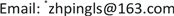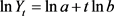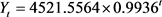﻿ 改进的指数曲线模型及其在西藏自治区水资源总量中的应用 An Improved Exponential Curve Model and its Application in the Total Water Resources in Tibet Autonomous Region

Operations Research and Fuzziology
Vol. 09  No. 04 ( 2019 ), Article ID: 32866 , 7 pages
10.12677/ORF.2019.94034

An Improved Exponential Curve Model and its Application in the Total Water Resources in Tibet Autonomous Region

Ping Zhang1*, Feng Luo2

1The Engineering & Technical College of Chengdu University of Technology, Department of Fundamental Education, Sichuan Leshan

2Leshan Vocational & Technical College, Department of Mechanical and Electrical Engineering, Sichuan LeshanReceived: Oct. 17th, 2019; accepted: Oct. 31st, 2019; published: Nov. 7th, 2019ABSTRACT

Based on the exponential curve model and the modified exponential curve model, an improved exponential curve model is proposed by introducing the time power term in this paper. According to the idea of non-linear least square method and trust region algorithm, the parameters of the new exponential curve model are estimated. On this basis, the model is applied to the total water resources of Tibet Autonomous Region. Firstly, the specific values of system parameters are solved by using the Matlab software, and then the specific expression of the new model is given. Furthermore, the results of the new model are compared with the classical exponential curve and the modified exponential curve model. The calculation results show that the new modified model has higher prediction accuracy on the total water resources in Tibet Autonomous Region.

Keywords:The Exponential Curve, Time Power Term, Non-Linear Least Squares Method, the Total Water Resources

1成都理工大学工程技术学院基础教学部，四川 乐山

2乐山职业技术学院机电工程系，四川 乐山1. 引言

2. 指数曲线和修正的指数曲线

2.1. 指数曲线

${Y}_{t}=a{b}^{t}$(1)(2)

$\left\{\begin{array}{l}\sum \mathrm{ln}Y=n\mathrm{ln}a+\left(\sum t\right)\mathrm{ln}b\\ \sum t\mathrm{ln}Y=\left(\sum t\right)\mathrm{ln}a+\left(\sum {t}^{2}\right)\mathrm{ln}b\end{array}$(3)

2.2. 修正指数曲线

${Y}_{t}=a{b}^{t}+K$(4)

${S}_{1}=\underset{t=0}{\overset{m-1}{\sum }}{Y}_{t}$${S}_{2}=\underset{t=m}{\overset{2m-1}{\sum }}{Y}_{t}$${S}_{2}=\underset{t=2m}{\overset{3m-1}{\sum }}{Y}_{t}$(5)

$\left\{\begin{array}{l}{S}_{1}=mK+a+ab+a{b}^{2}+\cdots +a{b}^{m-1}\\ {S}_{2}=mK+a{b}^{m}+a{b}^{m+1}+\cdots +a{b}^{2m-1}\\ {S}_{3}=mK+a{b}^{2m}+a{b}^{2m+1}+\cdots +a{b}^{3m-1}\end{array}$(6)

$\left\{\begin{array}{l}b={\left(\frac{{S}_{3}-{S}_{2}}{{S}_{2}-{S}_{1}}\right)}^{\frac{1}{m}}\\ a=\left({S}_{2}-{S}_{1}\right)\frac{b-1}{{\left({b}^{m}-1\right)}^{2}}\\ K=\frac{1}{m}\left({S}_{1}-\frac{a\left({b}^{m}-1\right)}{b-1}\right)\end{array}$(7)

3. 改进的幂次项指数曲线模型

${Y}_{t}=a{b}^{t}+c{t}^{\alpha }+d$(8)

$X=\left(a,b,c,d,\alpha \right)$$Y={\left({Y}_{1},{Y}_{2},\cdots ,{Y}_{n}\right)}^{\text{T}}$ 为观测值，则通过方程(8)可得到如下的非线性方程组

$\stackrel{^}{Y}=f\left(X\right)=\left[\begin{array}{c}ab+c+d\\ a{b}^{2}+c{2}^{\alpha }+d\\ ⋮\\ a{b}^{n}+c{n}^{\alpha }+d\end{array}\right]$(9)

$\mathrm{min}\begin{array}{c}\end{array}I=\underset{t=1}{\overset{n}{\sum }}\Delta {E}_{t}^{2}={‖f\left(\stackrel{^}{X}\right)-Y‖}^{2}={\left(f\left(\stackrel{^}{X}\right)-Y\right)}^{\text{T}}\left(f\left(\stackrel{^}{X}\right)-Y\right)$(10)

${Q}_{k}\left({d}_{k}\right)=I\left({\stackrel{^}{X}}_{k}\right){g}_{k}^{\text{T}}{d}_{k}+\frac{1}{2}{d}_{k}^{\text{T}}{H}_{k}{d}_{k}$(11)

$\begin{array}{l}{q}_{k}\left({d}_{k}\right)=I\left({\stackrel{^}{X}}_{k}\right){g}_{k}^{\text{T}}{d}_{k}+\frac{1}{2}{d}_{k}^{\text{T}}{H}_{k}{d}_{k}\\ \text{s}\text{.t}\text{.}\text{\hspace{0.17em}}\text{\hspace{0.17em}}\text{\hspace{0.17em}}‖{d}_{k}‖\le {\Delta }_{k}\end{array}$(12)

${g}_{k}=\left(\begin{array}{c}\begin{array}{c}{g}_{1}^{\left(k\right)},{g}_{2}^{\left(k\right)},{g}_{3}^{\left(k\right)},{g}_{4}^{\left(k\right)},{g}_{5}^{\left(k\right)}\end{array}\end{array}\right)={\left(\frac{\partial I}{\partial a},\frac{\partial I}{\partial b},\frac{\partial I}{\partial c},\frac{\partial I}{\partial d},\frac{\partial I}{\partial \alpha }\right)|}_{X={X}^{\left(k\right)}},k=0,1,\cdots$(13)

xk为第k次迭代点， ${d}_{k}={x}_{k+1}-{x}_{k}$ 为试探步， ${H}_{k}={\nabla }^{2}I\left({x}_{k}\right)$ 为Hess矩阵

${H}_{k}={\left[\begin{array}{ccccc}\frac{{\partial }^{2}I}{\partial {a}^{2}}& \frac{{\partial }^{2}I}{\partial ab}& \frac{{\partial }^{2}I}{\partial ac}& \frac{{\partial }^{2}I}{\partial ad}& \frac{{\partial }^{2}I}{\partial a\alpha }\\ \frac{{\partial }^{2}I}{\partial ba}& \frac{{\partial }^{2}I}{\partial {b}^{2}}& \frac{{\partial }^{2}I}{\partial bc}& \frac{{\partial }^{2}I}{\partial bd}& \frac{{\partial }^{2}I}{\partial b\alpha }\\ \frac{{\partial }^{2}I}{\partial ca}& \frac{{\partial }^{2}I}{\partial cb}& \frac{{\partial }^{2}I}{\partial {c}^{2}}& \frac{{\partial }^{2}I}{\partial cd}& \frac{{\partial }^{2}I}{\partial c\alpha }\\ \frac{{\partial }^{2}I}{\partial da}& \frac{{\partial }^{2}I}{\partial db}& \frac{{\partial }^{2}I}{\partial dc}& \frac{{\partial }^{2}I}{\partial {d}^{2}}& \frac{{\partial }^{2}I}{\partial d\alpha }\\ \frac{{\partial }^{2}I}{\partial \alpha a}& \frac{{\partial }^{2}I}{\partial \alpha b}& \frac{{\partial }^{2}I}{\partial \alpha c}& \frac{{\partial }^{2}I}{\partial \alpha d}& \frac{{\partial }^{2}I}{\partial {\alpha }^{2}}\end{array}\right]}_{X={X}^{\left(k\right)}},\text{\hspace{0.17em}}k=0,1,\cdots$ (14)

${\Delta }_{k}>0$ 为信赖域半径，其要在信赖域范围内， $‖.‖$ 为Euclid范数。

$Are{d}_{k}=I\left({\stackrel{^}{X}}_{k}\right)-I\left({\stackrel{^}{X}}_{k}+{d}_{k}\right)$(15)

$Pre{d}_{k}={Q}_{k}\left(0\right)-{Q}_{k}\left({d}_{k}\right)\text{=}-\left({g}_{k}^{\text{T}}{d}_{k}+\frac{1}{2}{d}_{k}^{\text{T}}{H}_{k}{d}_{k}\right)$(16)

${r}_{k}=\frac{Are{d}_{k}}{Pre{d}_{k}}$(17)

4. 具体应用Table 1. The raw data of the total water resources of tibet autonomous region (hundred million cubic meters)

$\text{APE}=|\frac{{Y}_{t}-{\stackrel{^}{Y}}_{t}}{{Y}_{t}}|×100%,t=1,2,\cdots ,n$(18)

$\text{MAPE}=\frac{1}{v-l+1}\underset{t=l}{\overset{v}{\sum }}|\frac{{Y}_{t}-{\stackrel{^}{Y}}_{t}}{{Y}_{t}}|×100%,v\le n$(19)(20)

${Y}_{t}=-0.0012×{2.9199}^{t}+4398.7213$(21)

${Y}_{t}=-4336.3911×{\left(-0.1743\right)}^{t}+14.3774×{t}^{1.6839}+3583.8882$(22)Table 2. The calculation results of the total water resources in Tibet autonomous region by using the exponential curve, modified exponential curve and improved exponential curve

5. 结论

An Improved Exponential Curve Model and its Application in the Total Water Resources in Tibet Autonomous Region[J]. 运筹与模糊学, 2019, 09(04): 292-298. https://doi.org/10.12677/ORF.2019.94034

1. 1. 唐五湘. 指数曲线模型参数估计的两步最小二乘法[J]. 预测, 1994(6): 68-69.

2. 2. 白雪梅, 赵松山. 对指数曲线模型参数估计方法的探讨[J]. 数量经济技术经济研究, 1997(10): 50-51.

3. 3. 杨桂元. 指数曲线预测模型的参数估计与误差分析[J]. 运筹与管理, 2003, 2(4): 55-58.

4. 4. 毛艺萍, 王斌会. 增长型曲线模型参数估计的新思路[J]. 统计与决策, 2006(8): 14-16.

5. 5. 秦尚林, 陈善雄, 许锡昌. 路基沉降预测的拓展指数曲线模型[J]. 铁道标准设计, 2010(2): 28-30.

6. 6. 张军, 吕雄, 姚贵平. 修正指数曲线模型参数估计的一种新方法[J]. 内蒙古农业大学学报(自然科学版), 2012(1): 285-290.

7. 7. 欧阳明, 丁伯阳, 石吉森. 单桩荷载-沉降曲线的修正指数曲线模型拟合研究[J]. 水运工程, 2013(1): 31-38.

8. 8. 陈希鸣, 黄张裕, 王睿, 秦洁, 刘仁志. 基于灰色GM(1,1)与指数曲线组合模型的高速公路沉降预测[J]. 勘察科学技术, 2019(1): 10-12.

9. 9. 贾俊平, 何晓群, 金勇进. 统计学(第7版)[M]. 北京: 中国人民大学出版社, 2019.

10. NOTES

*通讯作者。